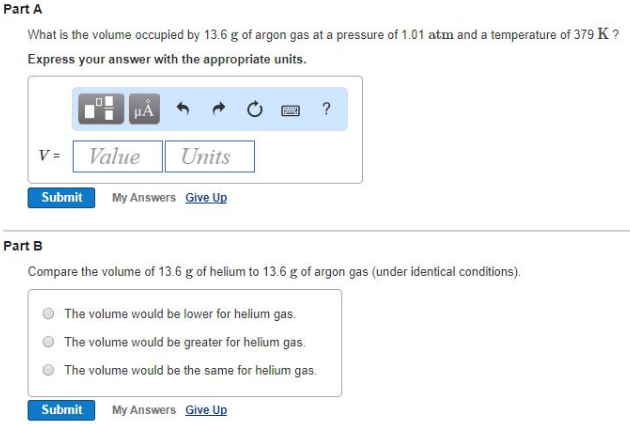# Problem: Part AWhat is the volume occupied by 13.6 g of argon gas at a pressure of 1.01 atm and a temperature of 379 K? Express your answer with the appropriate units. Part BCompare the volume of 13.6 g of helium to 13.6 g of argon gas (under identical conditions).           (a) The volume would be lower for helium gas.           (b) The volume would be greater for helium gas.           (c) The volume would be the same for helium gas.

###### FREE Expert Solution

Part A

95% (114 ratings)###### Problem Details

Part A

What is the volume occupied by 13.6 g of argon gas at a pressure of 1.01 atm and a temperature of 379 K?

Part B

Compare the volume of 13.6 g of helium to 13.6 g of argon gas (under identical conditions).

(a) The volume would be lower for helium gas.

(b) The volume would be greater for helium gas.

(c) The volume would be the same for helium gas.